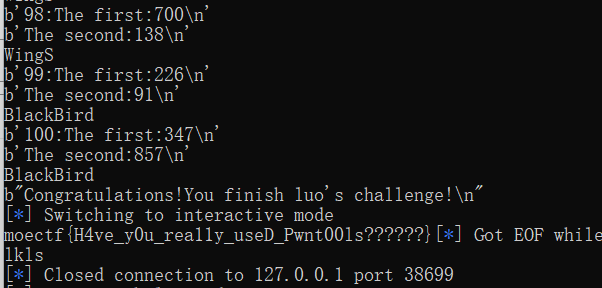# 初学pwn的一步一步解题学习的思路

``````Pwn主要学习语言会用到C语言和python语言，C用于读代码，python用于写脚本(exp)。

``````

from pwn import *
sh = remote("IP addr",port) //本地程勋就用sh = process("./程序名")

for i in range(100)：
//这两个函数就只是把信息接受到，不做任何处理，因为没啥用。接下来的是关键

numbers = s.split(b"+")
num0=int(numbers)

s = numbers.split(b"=")
num1=int(s)
num2=int(s)

if(num0+num1==num2):
sh.sendline(b'BlackBird')
print("BlackBird")
else:
sh.sendline(b'WingS')
print("WingS")

``````from pwn import *
sh = remote("127.0.0.1",40626)
for i in range(100):
print(ss)
print(ss)
numbers = s.split(b"+")
num0=int(numbers)
s = numbers.split(b"=")
num1=int(s)
num2=int(s)
if(num0+num1==num2):
sh.sendline(b'BlackBird')
print("BlackBird")
else:
sh.sendline(b'WingS')
print("WingS")